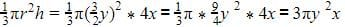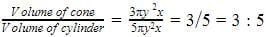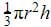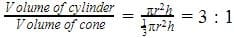Test: Volume Combination of Solids

# Test: Volume Combination of Solids

Test Description

## 20 Questions MCQ Test NCERT Mathematics for CAT Preparation | Test: Volume Combination of Solids

Test: Volume Combination of Solids for Class 10 2022 is part of NCERT Mathematics for CAT Preparation preparation. The Test: Volume Combination of Solids questions and answers have been prepared according to the Class 10 exam syllabus.The Test: Volume Combination of Solids MCQs are made for Class 10 2022 Exam. Find important definitions, questions, notes, meanings, examples, exercises, MCQs and online tests for Test: Volume Combination of Solids below.
Solutions of Test: Volume Combination of Solids questions in English are available as part of our NCERT Mathematics for CAT Preparation for Class 10 & Test: Volume Combination of Solids solutions in Hindi for NCERT Mathematics for CAT Preparation course. Download more important topics, notes, lectures and mock test series for Class 10 Exam by signing up for free. Attempt Test: Volume Combination of Solids | 20 questions in 20 minutes | Mock test for Class 10 preparation | Free important questions MCQ to study NCERT Mathematics for CAT Preparation for Class 10 Exam | Download free PDF with solutions
 1 Crore+ students have signed up on EduRev. Have you?
Test: Volume Combination of Solids - Question 1

### The radius of a cylindrical tank is 28m. If its capacity is equal to that of a rectangular tank of size 28mx16mx11m, then its depth is​

Detailed Solution for Test: Volume Combination of Solids - Question 1

Let d be the depth of the cylindrical tank. According to the given information, π(28)2 xd=28x16x11
⇒ d=2
Thus, the depth of the cylindrical tank is 2 m.

Test: Volume Combination of Solids - Question 2

### The ratio of the volume of a cube to that of a sphere which will exactly fit inside the cube is

Test: Volume Combination of Solids - Question 3

### A cone and a cylinder have their heights in the ratio 4: 5 and their diameters are in the ratio 3: 2. The ratio of their volumes will be​

Detailed Solution for Test: Volume Combination of Solids - Question 3

Height of cone =4x
Height of cylinder=5x
Diameter of cone=3y
Diameter of cylinder=2y
Volume of cone=Volume of cylinder= πr2h=y2*5xTest: Volume Combination of Solids - Question 4

Volume of a hollow right circular cylinder is​

Test: Volume Combination of Solids - Question 5

A container is in the form of a hemispherical bowl mounted by a hollow cylinder. The diameter of the sphere is 24 cm and the total height of the container is 16 cm. Its capacity is​

Test: Volume Combination of Solids - Question 6

The volume of a sphere (in cu. cm) is equal to its surface area (in sq. cm). The diameter of the sphere (in cm) is

Test: Volume Combination of Solids - Question 7

A solid toy is in the form of a right circular cylinder with a hemispherical shape at one end and a cone at the other end. Their common diameter is 4.2 cm and the heights of the cylindrical and conical portions are 12 cm and 7 cm respectively. The approximate volume of the toy will be​

Test: Volume Combination of Solids - Question 8

A cylinder, a cone and a hemisphere are of equal base and have the same height. The ratio of their volumes is​

Test: Volume Combination of Solids - Question 9

A spherical steel ball is melted to make 8 new identical balls. Then the radius of each new ball is how much times the radius of the original ball​

Test: Volume Combination of Solids - Question 10

A river 3 m deep and 10 m wide is flowing at the rate of 2 km per hour. How much water will fall into the sea in a minute?​

Test: Volume Combination of Solids - Question 11

A largest sphere is carved out of a cube of side 7cm. The volume of the sphere is​

Test: Volume Combination of Solids - Question 12

A cylinder whose height is two thirds of its diameter has the same volume as a sphere of radius 4 cm. The radius of the base of the cylinder will be​

Test: Volume Combination of Solids - Question 13

The radii of two right circular cylinders are in the ratio 2: 3 and their heights are in the ratio 5: 4. The ratio of their volumes will be

Test: Volume Combination of Solids - Question 14

A cylindrical tub of radius 5 cm and length 9.8 cm is full of water. A solid in the form of a right circular cone mounted on a hemisphere is immersed in the tub. If the radius of the a hemisphere is 3.5 cm and height of cone outside the hemisphere is 5 cm, find the volume of the water left in the tub.​

Test: Volume Combination of Solids - Question 15

The surface area of a cube is equal to the surface area of a sphere. The ratio of their volumes will be

Test: Volume Combination of Solids - Question 16

The volume of a largest sphere than can be cut from cylindrical log of wood of base radius 1 m and height 4 m is:

Test: Volume Combination of Solids - Question 17

A cylindrical vessel of diameter 42 cm and height 40 cm contains water up to a depth of 16 cm. Now a solid iron cylinder, with diameter 14 cm and height 32 cm is placed upright in the cylindrical vessel. The volume of the water required to just submerge the solid cylinder will be

Test: Volume Combination of Solids - Question 18

A rectangular paper is 44 cm long and 20 cm wide. A cylinder is formed by rolling the paper along its length. The volume of the cylinder will be​

Test: Volume Combination of Solids - Question 19

A cylinder, a cone and a hemisphere have equal base and height. Find the ratio of their volumes

Test: Volume Combination of Solids - Question 20

A cylinder and a cone are of same base radius and of same height. The ratio of the volume of cylinder to that of the cone is:​

Detailed Solution for Test: Volume Combination of Solids - Question 20

We have radius of cylinder and cone = r
Height of cone and cylinder = h
Volume of cone =Volume of cylinder = πr2h## NCERT Mathematics for CAT Preparation

276 docs|149 tests
 Use Code STAYHOME200 and get INR 200 additional OFF Use Coupon Code
Information about Test: Volume Combination of Solids Page
In this test you can find the Exam questions for Test: Volume Combination of Solids solved & explained in the simplest way possible. Besides giving Questions and answers for Test: Volume Combination of Solids, EduRev gives you an ample number of Online tests for practice

## NCERT Mathematics for CAT Preparation

276 docs|149 tests